# Global AI and Data Science

View Only

## Visualizing Geospatial Data in Python

####By Paco Nathan posted Sun May 17, 2020 11:25 PM

Throughout the global pandemic, many people have spent lots of time viewing maps that visualize data. Important data. People who work in data science are probably seeing increased needs to work with geospatial data, especially for visualizations. There are increased needs to understand metrics about geographic regions, to analyze supply chain, make plans that take into account local conditions and rules, etc.

This article shows how to use two popular geospatial libraries in Python:

• geopandas: extends Pandas to allow spatial operations on geometric types
• geoplot: a high-level geospatial plotting library

The second library is especially helpful since it builds on top of several other popular geospatial libraries, to simplify the coding that’s typically required. Those include: cartopy, which in turn leverages Cython, NumPy, GEOS, Shapely, pyshp, PROJ, Six, and perhaps a few others such as mapclassify, depending on which features you need to use.

Note: all of this code is available in a Jupyter notebook at github.com/DerwenAI/ibm_dsc_articles/blob/master/2020_05/tutorial.ipynb

## Installation

Installation should be quick. Just use the following three command lines with conda:

conda install -c conda-forge geopandas
conda install -c conda-forge geoplot
conda install pysal

Note: if you run into problems with these installations, there are alternative approaches available. Some additional notes discuss how to build these dependencies on Linux
github.com/DerwenAI/ibm_dsc_articles/blob/master/2020_05/INSTALL.md

## Terminology

Part of the learning curve for working with geospatial data is that there’s lots of special terminology used. Here’s a handy cheat-sheet for terms that you’re likely to encounter:

• shapefile: data file format used to represent items on a map
• geometry: a vector (generally a column in a dataframe) used to represent points, polygons, and other geometric shapes or locations, usually represented as well-known text (WKT)
• polygon: an area
• point: a specific location
• basemap: the background setting for a map, such as county borders in California
• projection: since the Earth is a 3D spheroid, chose a method for how an area gets flattened into 2D map, using some coordinate reference system (CRS)
• colormap: choice of a color palette for rendering data, selected with the cmap parameter
• overplotting: stacking several different plots on top of one another
• choropleth: using different hues to color polygons, as a way to represent data levels
• kernel density estimation: a data smoothing technique (KDE) that creates contours of shading to represent data levels
• cartogram: warping the relative area of polygons to represent data levels
• quantiles: binning data values into a specified number of equal-sized groups
• voronoi diagram: dividing an area into polygons such that each polygon contains exactly one generating point and every point in a given polygon is closer to its generating point than to any other; also called a Dirichlet tessellation

Okay, with those terms defined here for reference … let’s go!

## Get Some Data

We need to get some data to use for these examples. While geoplot includes plenty of sample datasets in the geojson format, it helps to know how to load your own data.

First, let’s get a shapefile from the US Census Bureau TIGER database to visualize state boundaries, which we’ll place into a maps subdirectory:

wget https://www2.census.gov/geo/tiger/GENZ2018/shp/cb_2018_us_state_20m.zip
mkdir maps
cd maps
unzip ../cb_2018_us_state_20m.zip

Of course, there are so many open data sources for shapefiles like this. Here are a few:

Next let’s get some data to plot, in this case the 2018 population estimates for the US, which we’ll place into a data subdirectory:

wget http://www2.census.gov/programs-surveys/popest/datasets/2010-2018/national/totals/nst-est2018-alldata.csv
mv nst-est2018-alldata.csv data

## Start Plotting

To import the required packages in Python:

import geoplot as gplt
import geopandas as gpd
import geoplot.crs as gcrs
import imageio
import pandas as pd
import pathlib
import matplotlib.pyplot as plt
import mapclassify as mc
import numpy as np

If you’re working in a
Jupyter notebook be sure to run the following “magic” command to render plots properly:

%matplotlib inline

Then to load a shapefile and view parts of it:Notice the
geometry column, which specifies the polygon shapes.

Now we’ll load the US Census data as a pandas dataframe and view a portion of it:

Next we
merge the shapefile with population data, joining on the state names:

pop_states = usa.merge(state_pop, left_on="NAME", right_on="NAME")

Great, now that data is ready to plot a shape. We’ll specify
California by name:

pop_states[pop_states.NAME=="California"].plot()Alternatively, we can create a
GeoDataFrame (a dataframe with geospatial data) by loading one of the sample datasets from geoplot, in this case the polygons for state boundaries:

path = gplt.datasets.get_path("contiguous_usa")

Then plot the map of the US states:

gplt.polyplot(contiguous_usa)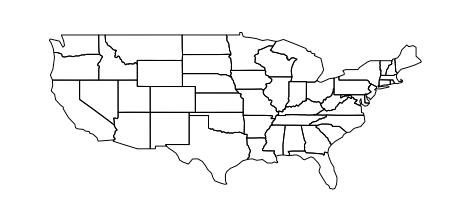Let’s load another sample dataset, in this case for US cities:

path = gplt.datasets.get_path("usa_cities")

Then plot the locations of each city in the continental US as points:

continental_usa_cities = usa_cities.query('STATE not in ["HI", "AK", "PR"]')
gplt.pointplot(continental_usa_cities)Composing these two, we’ll use overplotting to show the cities and states in the continental US. Note how the ax variable for the state polygons provides an axis onto which we plot the cities:

ax = gplt.polyplot(contiguous_usa)
gplt.pointplot(continental_usa_cities, ax=ax)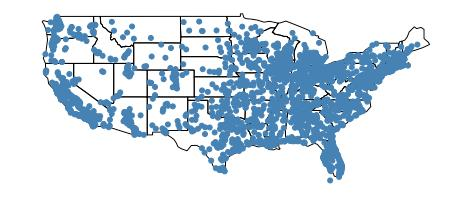That looks a bit stretched, so let’s adjust the projection to use an Albers equal-area conic projection:

ax = gplt.polyplot(contiguous_usa, projection=gcrs.AlbersEqualArea())
gplt.pointplot(continental_usa_cities, ax=ax)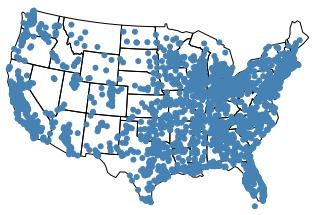Okay, that’s better! Again, since the Earth is a 3D globe, a projection is a method for how an area gets flattened into 2D map, using some coordinate reference system (CRS). The geoplot library makes this easy for us to use any number of projections – Albers equal-area projection is a choice in line with documentation from the libraries. You could also play with some you may remember from grade school like the mercator gcrs.Mercator() or modern variations of it like the gcrs.WebMercator()projection. Play around with them and let us know which ones you prefer and why!

## Representing Data

Now let’s compare several different ways to visualize geospatial data. First, we’ll change the hue of a city’s plotted point based on that city’s elevation, and also add a legend for people to decode the meaning of the different hues. The parameter lists start to get long-ish, so we’ll specify parameters on different lines:

ax = gplt.polyplot(contiguous_usa, projection=gcrs.AlbersEqualArea())
gplt.pointplot(
continental_usa_cities,
ax=ax,
hue="ELEV_IN_FT",
legend=True
)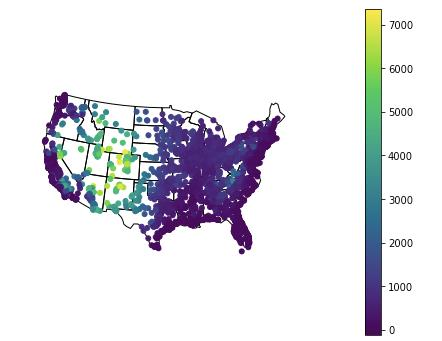We can also use the
scale of each plotted point to represent another dimension. In this case, the scale of the city points is based on their elevation:

ax = gplt.polyplot(
contiguous_usa,
edgecolor="white",
facecolor="lightgray",
figsize=(12, 8),
projection=gcrs.AlbersEqualArea()
)
gplt.pointplot(
continental_usa_cities,
ax=ax,
hue="ELEV_IN_FT",
cmap="Blues",
scheme="quantiles",
scale="ELEV_IN_FT",
limits=(1, 10),
legend=True,
legend_var="scale",
legend_kwargs={"frameon": False},
legend_values=[-110, 1750, 3600, 5500, 7400],
legend_labels=["-110 feet", "1750 feet", "3600 feet", "5500 feet", "7400 feet"]
)
ax.set_title("Cities in the continental US, by elevation", fontsize=16)With a
choropleth we use different hues to shade polygons, to represent a dimension of data:

ax = gplt.polyplot(contiguous_usa, projection=gcrs.AlbersEqualArea())
gplt.choropleth(
contiguous_usa,
hue="population",
edgecolor="white",
linewidth=1,
cmap="Greens",
legend=True,
scheme="FisherJenks",
legend_labels=[
"<3 million", "3-6.7 million", "6.7-12.8 million",
"12.8-25 million", "25-37 million"
],
projection=gcrs.AlbersEqualArea(),
ax=ax
)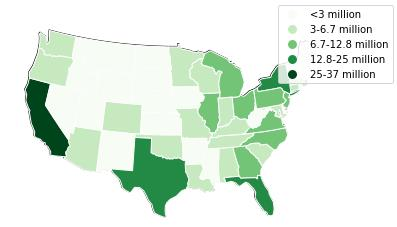A data smoothing technique known as kernel density estimation (KDE) creates contours to represent a dimension of data. In this case, we’ll zoom in to view the traffic collisions in the NYC boroughs:

ax = gplt.polyplot(boroughs, projection=gcrs.AlbersEqualArea())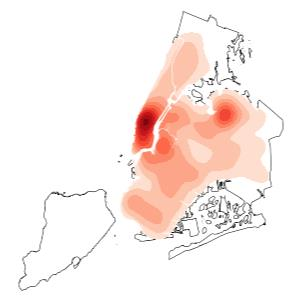Let’s zoom out to try KDE on major population centers throughout the US:

ax = gplt.polyplot(contiguous_usa, projection=gcrs.AlbersEqualArea())
gplt.kdeplot(
continental_usa_cities,
cmap="Reds",
clip=contiguous_usa,
ax=ax
)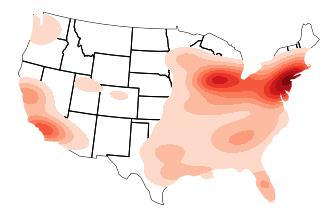This next section shows how to work with data associated with areas (polygons). We’ll load a dataset about obesity rates by US state:Convert that into a GeoDataFrame using a
join. Note how this adds the required geometry column:

geo_obesity = contiguous_usa.set_index("state").join(obesity.set_index("State"))Now we can use this data to plot a
cartogram, which grows or shrinks polygons to represent a dimension of data – in this case, the obesity rates per state:

gplt.cartogram(
geo_obesity,
scale="Percent",
projection=gcrs.AlbersEqualArea()
)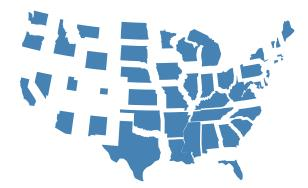One good approach to simplifying data visualization is binning the data into
quantiles. These are equal-sized groups, in this case 10 quantiles for elevation:

scheme = mc.Quantiles(continental_usa_cities["ELEV_IN_FT"], k=10)

Here we’ve divided the elevations into 10 quantiles with approximately 375 values each. Now let’s assign a different
hue to each quantile, plus a legend to explain them:

gplt.pointplot(
continental_usa_cities,
projection=gcrs.AlbersEqualArea(),
hue="ELEV_IN_FT",
scheme=scheme,
cmap="inferno_r",
legend=True
)Note how the
colormap was changed to the "inferno_r" setting.

Next, let’s add a filter for typical warnings that can be ignored:

import warnings
warnings.filterwarnings("ignore", "GeoSeries.isna", UserWarning)

The next example uses a
voronoi diagram, to calculate polygon areas based on a dimension of the data. Each polygon is centered on a generating point, such that every location in the polygon is closer to its generating point than to any other. This is helpful when you want to examine a column of data, to see if it may have any geospatial correlations.

In the following example, we’ll plot the locations primary schools in Melbourne, Australia, and use a voronoi diagram to show where they are concentrated:

melbourne_primary_schools = df.query('School_Type == "Primary"')
ax = gplt.voronoi(
melbourne_primary_schools,
clip=melbourne,
linewidth=0.5,
edgecolor="white",
projection=gcrs.Mercator()
)
gplt.polyplot(
melbourne,
edgecolor="None",
facecolor="lightgray",
ax=ax
)
gplt.pointplot(
melbourne_primary_schools,
color="black",
ax=ax,
s=1,
extent=melbourne.total_bounds
)
plt.title("Primary Schools in Greater Melbourne, 2018")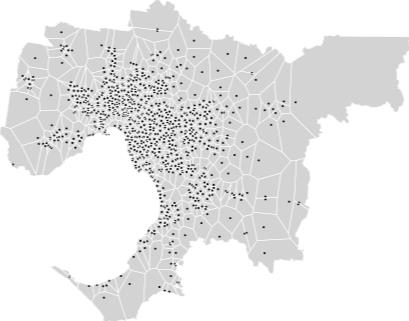Let’s construct a voronoi diagram for the elevations of US cities. This is a data smoothing technique since the elevations are for points, but we’ll “smooth” those values across areas:

proj = gplt.crs.AlbersEqualArea(
central_longitude=-98,
central_latitude=39.5
)
ax = gplt.voronoi(
continental_usa_cities,
hue="ELEV_IN_FT",
clip=contiguous_usa,
projection=proj,
cmap="Reds",
legend=True,
edgecolor="white",
linewidth=0.01
)
gplt.polyplot(
contiguous_usa,
ax=ax,
extent=contiguous_usa.total_bounds,
edgecolor="black",
linewidth=1,
zorder=1
)## Visualizing COVID-19 Data

Next, let’s download some of the COVID-19 data from the University of Washington’s IHME center. Change the name of the UNZIP’ed directory (download date) as needed:

wget https://ihmecovid19storage.blob.core.windows.net/latest/ihme-covid19.zip
unzip ihme-covid19.zip
mv 2020_*/Hospitalization_all_locs.csv data

We’ll filter rows, limiting this visualization to Earth Day (April 22) 2020:

is_earthday = ihme["date"]=="2020-04-22"

Now merge on state name with the continental US dataset from earlier:

cv19 = contiguous_usa.merge(ihme[is_earthday], left_on="state", right_on="location_name")

Add a calculated column for “deaths per million”:

deaths_per_mil = cv19["deaths_mean"] / cv19["population"] * 1000000.0
cv19["deaths_per_mil"] = deaths_per_mil

Then to visualize this data, let’s plot a choropleth of “deaths per million” per state, and overplot with population per city:

ax = gplt.choropleth(
cv19,
hue="deaths_per_mil",
edgecolor="white",
linewidth=5,
cmap="Reds",
alpha = 0.8,
projection=gcrs.AlbersEqualArea(),
figsize=(30, 30)
)
ax = gplt.pointplot(
continental_usa_cities,
hue="POP_2010",
cmap="Greens",
scheme="quantiles",
scale="POP_2010",
limits=(3, 50),
zorder=2,
alpha = 0.4,
ax=ax
)Note how the choropleth is overplotted with a point plot by reusing the same
ax variable. The point plot specifies a zorder parameter (which “layer”) and an alpha parameter (how “translucent”) to make the overplotting more readable. The figsize parameter in the first plot modifies the overall size of the plot.

This visualization shows where population centers in the US are more at risk: NYC area, Louisiana, Illinois, Michigan, Georgia, etc., which is where the news headlines in the US about COVID-19 on Earth Day 2020 date were focused.

## Animated Maps

Next, let’s prepare to animate this visualization. We’ll define a function to visualize the data for a given day:

def plot_choropleth (anim_path, date, cv19, cities):
ax = gplt.choropleth(
cv19,
hue="deaths_per_mil",
edgecolor="white",
linewidth=5,
cmap="Reds",
alpha = 0.8,
projection=gcrs.AlbersEqualArea(),
figsize=(30, 30)
)

ax = gplt.pointplot(
cities,
hue="POP_2010",
cmap="Greens",
scheme="quantiles",
scale="POP_2010",
limits=(3, 50),
zorder=2,
alpha = 0.4,
ax=ax
)
ax.set_title(
f"COVID-19 deaths/million vs. population on {date}",
fontsize=36
)
file_name = str(anim_path / "{}.png".format(date.replace("-", "")))
return file_name

Then define a range of dates:

date_set = set([])
for d in ihme["date"].tolist():
if d >= "2020-03-23" and d <= "2020-04-01":
dates = sorted(list(date_set))

Iterate through those dates, creating a visualization for each date, which gets saved to a file:

anim_path = pathlib.Path("anim/")
anim_path.mkdir(parents=True, exist_ok=True)
fig = plt.figure()
image_files = []
for date in dates:
is_earthday = ihme["date"]==date
cv19 = contiguous_usa.merge(ihme[is_earthday], left_on="state", right_on="location_name")

deaths_per_mil = cv19["deaths_mean"] / cv19["population"] * 1000000.0
cv19["deaths_per_mil"] = deaths_per_mil
file_name = plot_choropleth(anim_path, date, cv19, continental_usa_cities)
image_files.append(file_name)

Use
imageio to stitch these individual plots together into an animated GIF, at a rate of 2 frames/second:

images = []
for file_name in image_files:
gif_path = "movie.gif"
imageio.mimsave(gif_path, images, fps=2)

Here's a video of the resulting animation:

Note: there is also the matplotlib.animation API, which we could have used instead of imageio.  The former has some bugs in recent releases, while the latter has more general-purpose uses.

Again, all of this code is available in a Jupyter notebook at github.com/DerwenAI/ibm_dsc_articles/blob/master/2020_05/tutorial.ipynb

If you’d like to experiment with extending this visualization example, there are lots of related datasets are available at https://covidtracking.com/data

Co-author: William Roberts

#COVID19
#GlobalAIandDataScience
#GlobalDataScience
#Hands-on
#Hands-on-feature
#Python